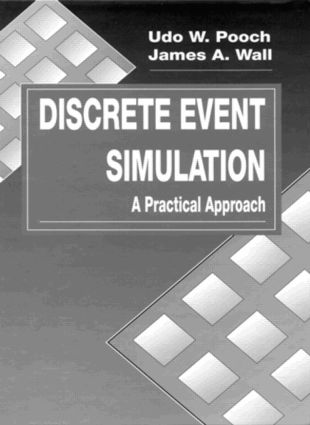# Discrete Event Simulation

## A Practical Approach, 1st Edition

CRC Press

432 pages

##### Purchasing Options:\$ = USD
Hardback: 9780849371745
pub: 1992-12-21
SAVE ~\$53.00
\$265.00
\$212.00
x

FREE Standard Shipping!

### Description

Discrete Event Simulation is a process-oriented text/reference that utilizes an eleven-step model to represent the simulation process from problem formulation to implementation and documentation. The book presents the necessary level of detail required to fully develop a model that produces meaningful results and considers the tools necessary to interpret those results. Sufficient background information is provided so that the underlying concepts of simulation are understood.

Major topics covered in Discrete Event Simulation include probability and distributional theory, statistical estimation and inference, the generation of random variates, verification and validation techniques, time management methods, experimental design, and programming language considerations. The book also examines distributed simulation and issues related to distributing the physical process over a network of tightly coupled processors. Topics covered in this area include deadlock, synchronization, rollback, event management, and communication processes.

Fully worked examples and numerous practical exercises have been drawn from the engineering disciplines and computer science, although they have been structured so that they will be useful as well to other disciplines such as economics, business administration, and management science. The presentation of techniques and methods in Discrete Event Simulation make it an ideal text/reference for all practitioners of discrete event simulation.

### Table of Contents

BASIC CONCEPTS AND TERMINOLOGY. Concept of a System. System Methodology. Advantages and Disadvantages of Simulation. Simulation Terminology. PROBABILITY CONCEPTS IN SIMULATION. Probability. Set Theory, Compound Events. Conditional Probability, Independent Events. Discrete Distributions. Continuous Distributions. RANDOM VARIABLES AND PROBABILITY DISTRIBUTIONS. Functions of a Random Variable. Moments. Generating Functions. Multivariate Distributions. DETAILED ANALYSIS OF COMMON PROBABILITY DISTRIBUTIONS. Bernoulli Distribution. Binomial Distribution. Geometric Distribution. Poisson Distribution. Uniform Distribution. Normal Distribution. Exponential Distribution. Chi-Square Distribution. Student's t-Distribution. F-Distribution. STATISTICS AND RANDOM SAMPLES. Descriptive Statistics and Frequency Diagrams. Statistics and Sampling Distributions. Method of Least Squares. Estimation. Confidence Interval Estimates. STATISTICAL TESTS. Tests of Hypotheses. Student's t-Test. The F-Test. The Chi-Square Goodness-of-Fit Test. The Kolmogorov-Smirnov Test. GENERATION OF RANDOM NUMBERS. Pseudorandom Numbers. Algorithms for Generating Pseudorandom Numbers. Testing and Validating Pseudorandom Sequences. Generation of Nonuniform Variates. DISCRETE SYSTEM SIMULATION. Simulation Terminology. Time Management Methods. Object Generation. Queue Management and List Processing. Collecting and Recording Simulation Data. MODEL VALIDATION. Evaluation of the Simulation Model. Validation Description. Sampling Methods. THE DESIGN OF SIMULATION EXPERIMENTS. Completely Randomized Design. Randomized Complete Block Design. Factorial Design. Network Simulation Model Performance Analysis. ESTIMATION OF MODEL PARAMETERS. Optimization of Response Surfaces. Heuristic Search. Complete Enumeration. Random Search. Steepest Ascent (Descent). Coordinate or Single-Variable Search. Parallel Tangent Search. Conjugate Direction Search. OUTPUT ANALYSIS. Analysis of Simulation Results. Estimation and Confidence Limits. Initial Conditions and Inputs. Simulation Model Run Length. Variance Reduction. LANGUAGES FOR DISCRETE SYSTEM SIMULATION. Language Characteristics. Use of Multipurpose Languages. Simulation Languages. DISTRIBUTED SIMULATION. The System Simulation Problem. Decomposition of a Simulation. Synchronization of Distributed Model Components. Deadlock Resolution in Distributed Simulations. QUEUEING THEORY AND SIMULATION. Review of the Poisson and Exponential Distributions. The M/M/1/8/FIFO System. Summary Measures for the M/M/1/8/FIFO System. The M/M/1/K/FIFO System. The M/M/C/8/FIFO System. Priority Queueing Systems. APPENDIX TABLES. Normal Distribution Function. Student's t-Distribution Function. Chi-Square Distribution Function. F-Distribution Function. Poisson Distribution Function. Critical Values for the Kolmogorov-Smirnov Test. INDEX.

### Subject Categories

##### BISAC Subject Codes/Headings:
COM046000
COMPUTERS / Operating Systems / General
COM059000
COMPUTERS / Computer Engineering